# Vision augmentation

Transforms to apply data augmentation in Computer Vision
``img = PILImage(PILImage.create(TEST_IMAGE).resize((600,400)))``

## RandTransform-

source

### RandTransform

`````` RandTransform (p:float=1.0, nm:str=None, before_call:callable=None,
**kwargs)``````

A transform that before_call its state at each `__call__`

Type Default Details
p float 1.0 Probability of applying Transform
nm str None
before_call callable None Optional batchwise preprocessing function
kwargs

As for all `Transform` you can pass `encodes` and `decodes` at init or subclass and implement them. You can do the same for the `before_call` method that is called at each `__call__`. Note that to have a consistent state for inputs and targets, a `RandTransform` must be applied at the tuple level.

By default the before_call behavior is to execute the transform with probability `p` (if subclassing and wanting to tweak that behavior, the attribute `self.do`, if it exists, is looked for to decide if the transform is executed or not).

Note

A `RandTransform` is only applied to the training set by default, so you have to pass `split_idx=0` if you are calling it directly and not through a `Datasets`. That behavior can be changed by setting the attr `split_idx` of the transform to `None`.

``RandTransform.before_call``
``<function __main__.RandTransform.before_call(self, b, split_idx: 'int')>``

source

### RandTransform.before_call

`` RandTransform.before_call (b, split_idx:int)``

This function can be overridden. Set `self.do` based on `self.p`

Type Details
b
split_idx int Index of the train/valid dataset
``````def _add1(x): return x+1
start,d1,d2 = 2,False,False
for _ in range(40):
t = dumb_tfm(start, split_idx=0)
if dumb_tfm.do: test_eq(t, start+1); d1=True
else:           test_eq(t, start)  ; d2=True
assert d1 and d2
dumb_tfm``````
``````_add1 -- {'p': 0.5}:

## Item transforms

source

### FlipItem

`` FlipItem (p:float=0.5)``

Randomly flip with probability `p`

Calls `@patch`’d `flip_lr` behaviors for `Image`, `TensorImage`, `TensorPoint`, and `TensorBBox`

``````tflip = FlipItem(p=1.)
test_eq(tflip(bbox,split_idx=0), tensor([[1.,0., 0.,1]]) -1)``````

source

### DihedralItem

`````` DihedralItem (p:float=1.0, nm:str=None, before_call:callable=None,
**kwargs)``````

Randomly flip with probability `p`

Type Default Details
p float 1.0 Probability of applying Transform
nm str None
before_call callable None Optional batchwise preprocessing function
kwargs

Calls `@patch`’d `dihedral` behaviors for `PILImage`, `TensorImage`, `TensorPoint`, and `TensorBBox`

By default each of the 8 dihedral transformations (including noop) have the same probability of being picked when the transform is applied. You can customize this behavior by passing your own `draw` function. To force a specific flip, you can also pass an integer between 0 and 7.

``````_,axs = subplots(2, 4)
for ax in axs.flatten():
show_image(DihedralItem(p=1.)(img, split_idx=0), ctx=ax)``````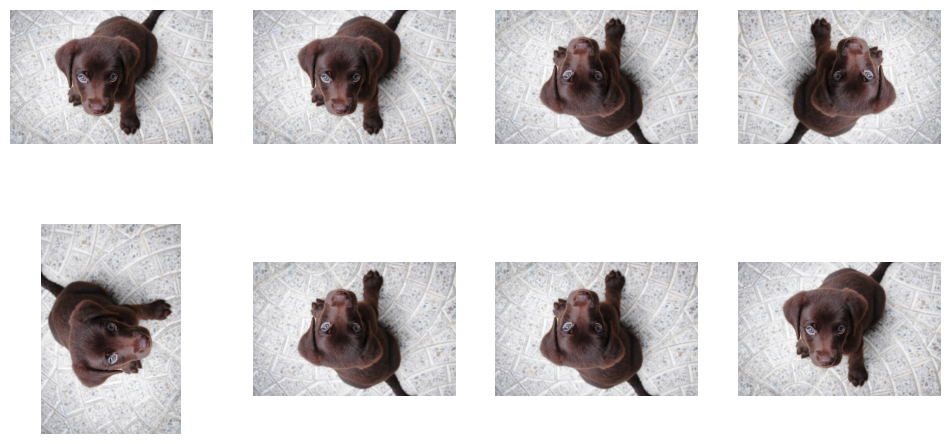## Resize with crop, pad or squish

`` PadMode (*args, **kwargs)``

All possible padding mode as attributes to get tab-completion and typo-proofing

source

`` CropPad (size:int|tuple, pad_mode:PadMode='zeros', **kwargs)``

Center crop or pad an image to `size`

Type Default Details
size int | tuple Size to crop or pad to, duplicated if one value is specified
pad_mode PadMode zeros A `PadMode`

Calls `@patch`’d `crop_pad` behaviors for `Image`, `TensorImage`, `TensorPoint`, and `TensorBBox`

``````_,axs = plt.subplots(1,3,figsize=(12,4))
for ax,sz in zip(axs.flatten(), [300, 500, 700]):
``````(300, 300)
(500, 500)
(700, 700)``````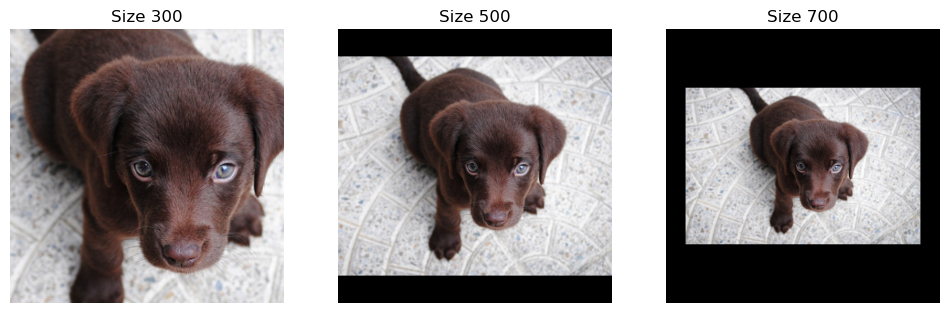``````_,axs = plt.subplots(1,3,figsize=(12,4))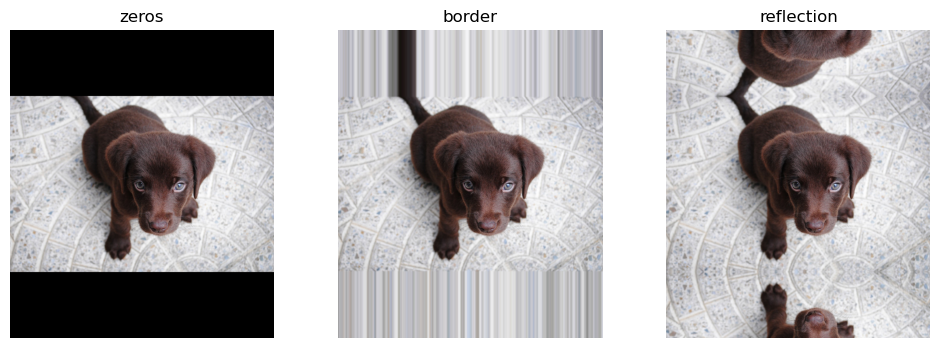source

### RandomCrop

`` RandomCrop (size:int|tuple, **kwargs)``

Randomly crop an image to `size`

Type Details
size int | tuple Size to crop to, duplicated if one value is specified

source

### OldRandomCrop

`````` OldRandomCrop (size:int|tuple, pad_mode:PadMode='zeros', enc=None,
dec=None, split_idx=None, order=None)``````

Randomly crop an image to `size`

Type Default Details
size int | tuple Size to crop or pad to, duplicated if one value is specified
pad_mode PadMode zeros A `PadMode`
enc NoneType None
dec NoneType None
split_idx NoneType None
order NoneType None
``````_,axs = plt.subplots(1,3,figsize=(12,4))
f = RandomCrop(200)
for ax in axs: show_image(f(img), ctx=ax);``````On the validation set, we take a center crop.

``````_,axs = plt.subplots(1,3,figsize=(12,4))
for ax in axs: show_image(f(img, split_idx=1), ctx=ax);``````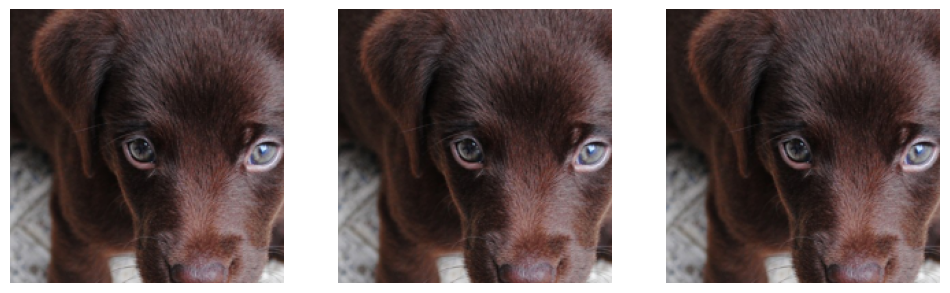### ResizeMethod

`` ResizeMethod (*args, **kwargs)``

All possible resize method as attributes to get tab-completion and typo-proofing

``test_eq(ResizeMethod.Squish, 'squish')``

source

### Resize

`````` Resize (size:int|tuple, method:ResizeMethod='crop',
2>, <Resampling.NEAREST: 0>), **kwargs)``````

A transform that before_call its state at each `__call__`

Type Default Details
size int | tuple Size to resize to, duplicated if one value is specified
method ResizeMethod crop A `ResizeMethod`
pad_mode PadMode reflection A `PadMode`
resamples tuple (<Resampling.BILINEAR: 2>, <Resampling.NEAREST: 0>) Pillow `Image` resamples mode, resamples for mask

`size` can be an integer (in which case images will be resized to a square) or a tuple. Depending on the `method`: - we squish any rectangle to `size` - we resize so that the shorter dimension is a match and use padding with `pad_mode` - we resize so that the larger dimension is match and crop (randomly on the training set, center crop for the validation set)

When doing the resize, we use `resamples` for images and `resamples` for segmentation masks.

``````_,axs = plt.subplots(1,3,figsize=(12,4))
for ax,method in zip(axs.flatten(), [ResizeMethod.Squish, ResizeMethod.Pad, ResizeMethod.Crop]):
rsz = Resize(256, method=method)
show_image(rsz(img, split_idx=0), ctx=ax, title=method);``````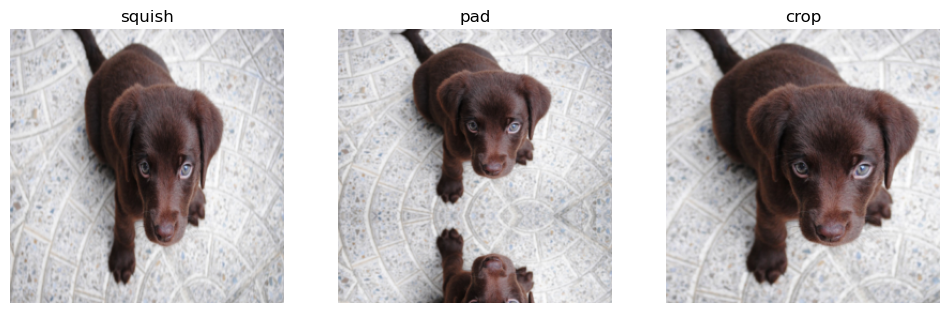On the validation set, the crop is always a center crop (on the dimension that’s cropped).

``````_,axs = plt.subplots(1,3,figsize=(12,4))
for ax,method in zip(axs.flatten(), [ResizeMethod.Squish, ResizeMethod.Pad, ResizeMethod.Crop]):
rsz = Resize(256, method=method)
show_image(rsz(img, split_idx=1), ctx=ax, title=method);``````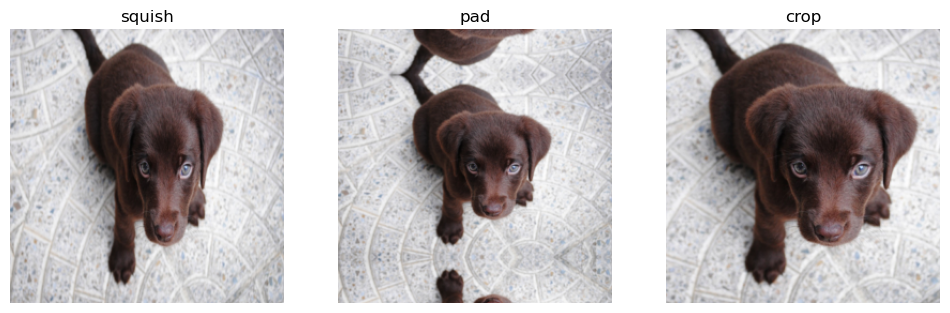source

### RandomResizedCrop

`````` RandomResizedCrop (size:int|tuple, min_scale:float=0.08, ratio=(0.75,
1.3333333333333333), resamples=(<Resampling.BILINEAR:
2>, <Resampling.NEAREST: 0>), val_xtra:float=0.14,
max_scale:float=1.0, **kwargs)``````

Picks a random scaled crop of an image and resize it to `size`

Type Default Details
size int | tuple Final size, duplicated if one value is specified,,
min_scale float 0.08 Minimum scale of the crop, in relation to image area
ratio tuple (0.75, 1.3333333333333333) Range of width over height of the output
resamples tuple (<Resampling.BILINEAR: 2>, <Resampling.NEAREST: 0>) Pillow `Image` resample mode, resamples for mask
val_xtra float 0.14 The ratio of size at the edge cropped out in the validation set
max_scale float 1.0 Maximum scale of the crop, in relation to image area

The crop picked as a random scale in range `(min_scale,max_scale)` and `ratio` in the range passed, then the resize is done with `resamples` for images and `resamples` for segmentation masks. On the validation set, we center crop the image if it’s ratio isn’t in the range (to the minmum or maximum value) then resize.

``````crop = RandomResizedCrop(256)
_,axs = plt.subplots(3,3,figsize=(9,9))
for ax in axs.flatten():
cropped = crop(img)
show_image(cropped, ctx=ax);``````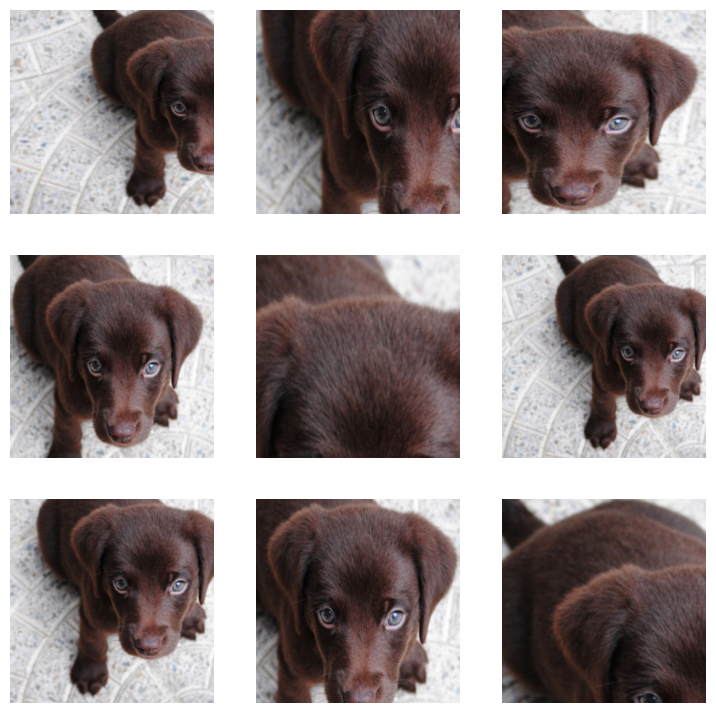``test_eq(cropped.shape, [256,256])``

Squish is used on the validation set, removing `val_xtra` proportion of each side first.

``````_,axs = subplots(1,3)
for ax in axs.flatten(): show_image(crop(img, split_idx=1), ctx=ax);``````By setting `max_scale` to lower values, one can enforce small crops.

``````small_crop = RandomResizedCrop(256, min_scale=0.05, max_scale=0.15)
_,axs = plt.subplots(3,3,figsize=(9,9))
for ax in axs.flatten():
cropped = small_crop(img)
show_image(cropped, ctx=ax);``````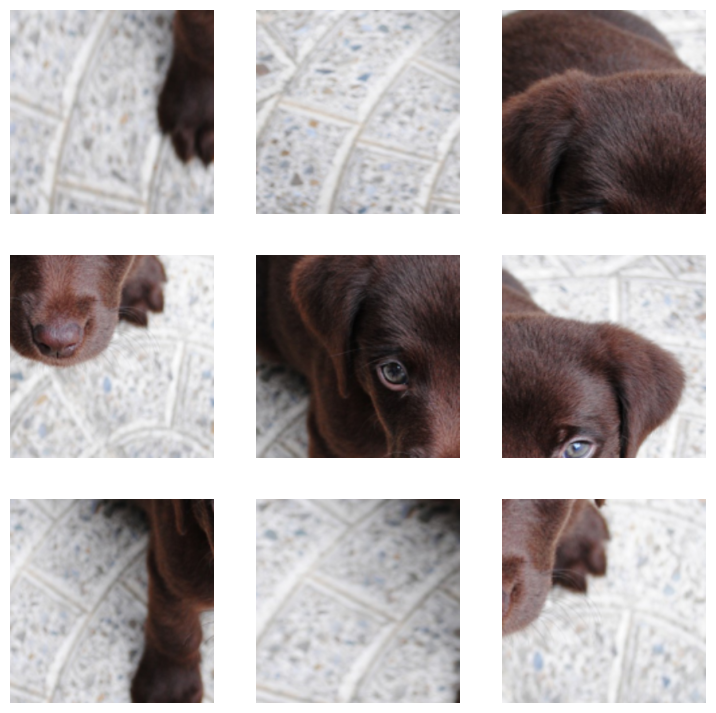source

### RatioResize

`````` RatioResize (max_sz:int, resamples=(<Resampling.BILINEAR: 2>,
<Resampling.NEAREST: 0>), **kwargs)``````

Resizes the biggest dimension of an image to `max_sz` maintaining the aspect ratio

Type Default Details
max_sz int Biggest dimension of the resized image
resamples tuple (<Resampling.BILINEAR: 2>, <Resampling.NEAREST: 0>) Pillow `Image` resample mode, resamples for mask
kwargs
``RatioResize(256)(img)``## Affine and coord tfm on the GPU

``````timg = TensorImage(array(img)).permute(2,0,1).float()/255.
def _batch_ex(bs): return TensorImage(timg[None].expand(bs, *timg.shape).clone())``````

Uses coordinates in `coords` to map coordinates in `x` to new locations for transformations such as `flip`. Preferably use `TensorImage.affine_coord` as this combines `_grid_sample` with `F.affine_grid` for easier usage. Use`F.affine_grid` to make it easier to generate the `coords`, as this tends to be large `[H,W,2]` where `H` and `W` are the height and width of your image `x`.

This is the image we start with, and are going to be using for the following examples.

``````img=torch.tensor([[[0,0,0],[1,0,0],[2,0,0]],
[[0,1,0],[1,1,0],[2,1,0]],
[[0,2,0],[1,2,0],[2,2,0]]]).permute(2,0,1)[None]/2.
show_images(img)``````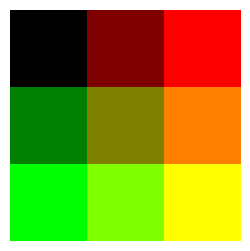Here we `_grid_sample`, but do not change the original image. Notice how the coordinates in `grid` map to the coordiants in `img`.

``````grid=torch.tensor([[[[-1,-1],[0,-1],[1,-1]],
[[-1,0],[0,0],[1,0]],
[[-1,1],[0,1],[1,1.]]]])
img=_grid_sample(img, grid,align_corners=True)
show_images(img)``````Next we do a flip by manually editing the grid.

``````grid=torch.tensor([[[1.,-1],[0,-1],[-1,-1]],
[[1,0],[0,0],[-1,0]],
[[1,1],[0,1],[-1,1]]])
img=_grid_sample(img, grid[None],align_corners=True)
show_images(img)``````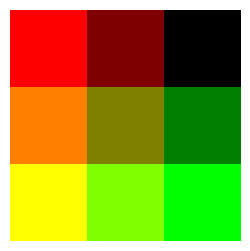Next we shift the image up by one. By default `_grid_sample` uses reflection padding.

``````grid=torch.tensor([[[[-1,0],[0,0],[1,0]],
[[-1,1],[0,1],[1,1]],
[[-1,2],[0,2],[1,2.]]]])
img=_grid_sample(img, grid,align_corners=True)
show_images(img)``````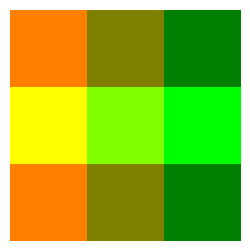`affine_coord` allows us to much more easily work with images, by allowing us to specify much smaller `mat`, by comparison to grids, which require us to specify values for every pixel.

source

### affine_grid

`` affine_grid (theta:torch.Tensor, size:tuple, align_corners:bool=None)``

Generates `TensorFlowField` from a transformation affine matrices `theta`

Type Default Details
theta Tensor Batch of affine transformation matrices
size tuple Output size
align_corners bool None PyTorch `F.grid_sample` align_corners

source

### AffineCoordTfm

`````` AffineCoordTfm (aff_fs:callable|MutableSequence=None,
coord_fs:callable|MutableSequence=None,
size:int|tuple=None, mode='bilinear',
align_corners=None, **kwargs)``````

Combine and apply affine and coord transforms

Type Default Details
aff_fs callable | MutableSequence None Affine transformations function for a batch
coord_fs callable | MutableSequence None Coordinate transformations function for a batch
size int | tuple None Output size, duplicated if one value is specified
mode str bilinear PyTorch `F.grid_sample` interpolation
pad_mode str reflection A `PadMode`
align_corners NoneType None PyTorch `F.grid_sample` align_corners
kwargs

Calls `@patch`’d `affine_coord` behaviors for `TensorImage`, `TensorMask`, `TensorPoint`, and `TensorBBox`

Multiplies all the matrices returned by `aff_fs` before doing the corresponding affine transformation on a basic grid corresponding to `size`, then applies all `coord_fs` on the resulting flow of coordinates before finally doing an interpolation with `mode` and `pad_mode`.

Here are examples of how to use `affine_coord` on images. Including the identity or original image, a flip, and moving the image to the left.

``````imgs=_batch_ex(3)
identity=torch.tensor([[1,0,0],[0,1,0.]])
flip=torch.tensor([[-1,0,0],[0,1,0.]])
translation=torch.tensor([[1,0,1.],[0,1,0]])
mats=torch.stack((identity,flip,translation))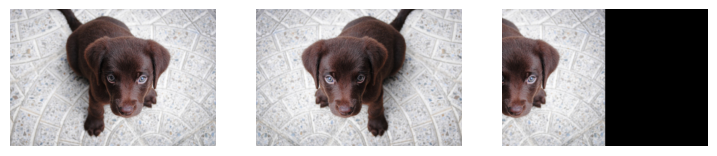Now you may be asking, “What is this `mat`”? Well lets take a quick look at the identify below.

``````imgs=_batch_ex(1)
identity=torch.tensor([[1,0,0],[0,1,0.]])
eye=identity[:,0:2]
bi=identity[:,2:3]
eye,bi``````
``````(tensor([[1., 0.],
[0., 1.]]),
tensor([[0.],
[0.]]))``````

Notice the tensor ‘eye’ is an identity matrix. If we multiply this by a single coordinate in our original image x,y we will simply the same values returned for x and y. bi is added after this multiplication. For example, lets flip the image so the left top corner is in the right top corner:

``````t=torch.tensor([[-1,0,0],[0,1,0.]])
eye=t[:,0:2]
bi=t[:,2:3]
xy=torch.tensor([-1.,-1]) #upper left corner
torch.sum(xy*eye,dim=1)+bi #now the upper right corner``````
``tensor([ 1., -1.])``

source

### AffineCoordTfm.compose

`` AffineCoordTfm.compose (tfm)``

Compose `self` with another `AffineCoordTfm` to only do the interpolation step once

source

### RandomResizedCropGPU

`````` RandomResizedCropGPU (size, min_scale=0.08, ratio=(0.75,
1.3333333333333333), mode='bilinear',
valid_scale=1.0, max_scale=1.0,

Picks a random scaled crop of an image and resize it to `size`

Type Default Details
size Final size, duplicated if one value is specified
min_scale float 0.08 Minimum scale of the crop, in relation to image area
ratio tuple (0.75, 1.3333333333333333) Range of width over height of the output
mode str bilinear PyTorch `F.grid_sample` interpolation
valid_scale float 1.0 Scale of the crop for the validation set, in relation to image area
max_scale float 1.0 Maximum scale of the crop, in relation to image area
mode_mask str nearest Interpolation mode for `TensorMask`
kwargs
``````t = _batch_ex(8)
rrc = RandomResizedCropGPU(224, p=1.)
y = rrc(t)
_,axs = plt.subplots(2,4, figsize=(12,6))
for ax in axs.flatten():
show_image(y[i], ctx=ax)``````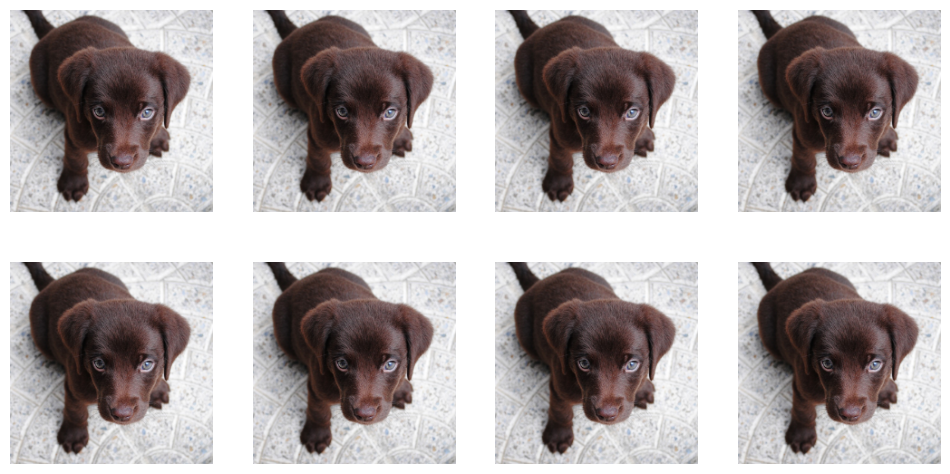Note

`RandomResizedCropGPU` uses the same region for all images in the batch.

### GPU helpers

This section contain helpers for working with augmentations on GPUs that is used throughout the code.

source

`` mask_tensor (x:torch.Tensor, p=0.5, neutral=0.0, batch=False)``

Mask elements of `x` with `neutral` with probability `1-p`

Type Default Details
x Tensor Input `Tensor`
p float 0.5 Probability of not applying mask
batch bool False Apply identical mask to entire batch

Lets look at some examples of how `mask_tensor` might be used, we are using `clone()` because this operation overwrites the input. For this example lets try using degrees for rotating an image.

``````with no_random():
x=torch.tensor([60,-30,90,-210,270,-180,120,-240,150])
``````p=0.5:  tensor([  60,  -30,   90, -210,    0, -180,    0,    0,  150])
p=1.0:  tensor([  60,  -30,   90, -210,  270, -180,  120, -240,  150])
p=0.0:  tensor([0, 0, 0, 0, 0, 0, 0, 0, 0])``````

Notice how `p` controls how likely a value is expected to be replaced with 0, or be unchanged since a 0 degree rotation would just be the original image. `batch` acts on the entire batch instead of single elements of the batch. Now lets consider a different example, of working with brightness. Note: with brightness 0 is a completely black image.

``````x=torch.tensor([0.6,0.4,0.3,0.7,0.4])
``````p=0.:  tensor([0., 0., 0., 0., 0.])
p=0.,neutral=0.5:  tensor([0.5000, 0.5000, 0.5000, 0.5000, 0.5000])``````

Here is would be very bad if we had a completely black image, as that is not an unchanged image. Instead we set `neutral` to 0.5 which is the value for an unchanged image for brightness.

`_draw_mask` is used to support the api of many following transformations to create `mask_tensor`s. (`p, neutral, batch`) are passed down to `mask_tensor`. `def_draw` is the default draw function, and what should happen if no custom user setting is provided. `draw` is user defined behavior and can be a function, list of floats, or a float. `draw` and `def_draw` must return a tensor.

Here we use random integers from 1 to 8 for our `def_draw`, this example is very similar to `Dihedral`.

``````x = torch.zeros(10,2,3)
def def_draw(x):
x=torch.randint(1,8, (x.size(0),))
return x
with no_random(): print(torch.randint(1,8, (x.size(0),)))
``````tensor([2, 3, 5, 6, 5, 4, 6, 6, 1, 1])
TensorBase([2, 0, 0, 6, 5, 4, 6, 0, 0, 1])``````

Next, there are three ways to define `draw`, as a constant, as a list, and as a function. All of these override `def_draw`, so that it has no effect on the final result.

``````with no_random():
print('list : ', _draw_mask(x, def_draw, draw=[1, 2, 3, 4, 5, 6, 7, 8, 9, 10]))
print('list : ',_draw_mask(x[0:2], def_draw, draw=[1, 2, 3, 4, 5, 6, 7, 8, 9, 10, 11, 12, 13, 14]))
print('funct: ',_draw_mask(x, def_draw, draw=lambda x: torch.arange(1,x.size(0)+1)))
try:
except AssertionError as e:
print(type(e),'\n',e)``````
``````const:  TensorBase([1., 1., 1., 1., 0., 1., 0., 0., 1., 1.])
list :  TensorBase([ 1.,  2.,  0.,  0.,  5.,  0.,  7.,  0.,  0., 10.])
list :  TensorBase([1., 0.])
funct:  TensorBase([ 1,  2,  3,  4,  0,  6,  7,  8,  9, 10])
<class 'AssertionError'>
``````

Note, when using a list it can be larger than the batch size, but it cannot be smaller than the batch size. Otherwise there would not be enough augmentations for elements of the batch.

``````x = torch.zeros(5,2,3)
def_draw = lambda x: torch.randint(0,8, (x.size(0),))
assert (0. <= t).all() and (t <= 7).all()
assert (0. <= t).all() and (t <= 1).all()
for i in range(5):
assert (t==torch.zeros(5)).all() or (t==torch.ones(5)).all()``````

#### Flip/Dihedral GPU Helpers

`affine_mat` is used to transform the length-6 vestor into a [bs,3,3] tensor. This is used to allow us to combine affine transforms.

source

### affine_mat

`` affine_mat (*ms)``

Restructure length-6 vector `ms` into an affine matrix with 0,0,1 in the last line

Here is an example of how flipping an image would look using `affine_mat`.

``````flips=torch.tensor([-1,1,-1])
ones=t1(flips)
zeroes=t0(flips)
affines=affine_mat(flips,zeroes,zeroes,zeroes,ones,zeroes)
print(affines)``````
``````tensor([[[-1,  0,  0],
[ 0,  1,  0],
[ 0,  0,  1]],

[[ 1,  0,  0],
[ 0,  1,  0],
[ 0,  0,  1]],

[[-1,  0,  0],
[ 0,  1,  0],
[ 0,  0,  1]]])``````

This is done so that we can combine multiple affine transformations without doing the math on the entire image. We need the matrices to be the same size, so we can do a matric multiple in order to combines affine transformations. While this is usually done on an entire batch, here is what it would look like to have multiple flip transformations for a single image. Since we flip twice we end up with an affine matrix that would simply return our original image.

If you would like more information on how this works, see `affine_coord`.

``````x = torch.eye(3,dtype=torch.int64)
for affine in affines:
x @= affine
print(x)``````
``````tensor([[-1,  0,  0],
[ 0,  1,  0],
[ 0,  0,  1]])
tensor([[-1,  0,  0],
[ 0,  1,  0],
[ 0,  0,  1]])
tensor([[1, 0, 0],
[0, 1, 0],
[0, 0, 1]])``````

`flip_mat` will generate a [bs,3,3] tensor representing our flips for a batch with probability `p`. `draw` can be used to define a function, constant, or list that defines what flips to use. If draw is a list, the length must be greater than or equal to the batch size. For `draw` 0 is the original image, or 1 is a flipped image. `batch` will mean that the entire batch will be flipped or not.

source

### flip_mat

Return a random flip matrix

Below are some examples of how to use draw as a constant, list and function.

``````with no_random():
x=torch.randn(2,4,3)
print('const: ',flip_mat(x, draw=1))
print('list : ', flip_mat(x, draw=[1, 0]))
print('list : ',flip_mat(x[0:2], draw=[1, 0, 1, 0, 1]))
print('funct: ',flip_mat(x, draw=lambda x: torch.ones(x.size(0))))
test_fail(lambda: flip_mat(x, draw=))``````
``````const:  TensorBase([[[-1.,  0.,  0.],
[ 0.,  1.,  0.],
[ 0.,  0.,  1.]],

[[ 1.,  0.,  0.],
[ 0.,  1.,  0.],
[ 0.,  0.,  1.]]])
list :  TensorBase([[[-1.,  0.,  0.],
[ 0.,  1.,  0.],
[ 0.,  0.,  1.]],

[[ 1.,  0.,  0.],
[ 0.,  1.,  0.],
[ 0.,  0.,  1.]]])
list :  TensorBase([[[-1.,  0.,  0.],
[ 0.,  1.,  0.],
[ 0.,  0.,  1.]],

[[ 1.,  0.,  0.],
[ 0.,  1.,  0.],
[ 0.,  0.,  1.]]])
funct:  TensorBase([[[ 1.,  0.,  0.],
[ 0.,  1.,  0.],
[ 0.,  0.,  1.]],

[[-1.,  0.,  0.],
[ 0.,  1.,  0.],
[ 0.,  0.,  1.]]])``````
``````x = flip_mat(torch.randn(100,4,3))
test_eq(set(x[:,0,0].numpy()), {-1,1}) #might fail with probability 2*2**(-100) (picked only 1s or -1s)``````

Flip images,masks,points and bounding boxes horizontally. `p` is the probability of a flip being applied. `draw` can be used to define custom flip behavior.

source

### Flip

`````` Flip (p=0.5, draw:int|MutableSequence|callable=None, size:int|tuple=None,
batch=False)``````

Randomly flip a batch of images with a probability `p`

Type Default Details
p float 0.5 Probability of applying flip
draw int | MutableSequence | callable None Custom flips instead of random
size int | tuple None Output size, duplicated if one value is specified
mode str bilinear PyTorch `F.grid_sample` interpolation
pad_mode str reflection A `PadMode`
align_corners bool True PyTorch `F.grid_sample` align_corners
batch bool False Apply identical flip to entire batch

Calls `@patch`’d `flip_batch` behaviors for `TensorImage`, `TensorMask`, `TensorPoint`, and `TensorBBox`

Here are some examples of using flip. Notice that a constant `draw=1`, is effectively the same as the default settings. Also notice the fine-tune control we can get in the third example, by setting `p=1.` and defining a custom draw.

``````with no_random(32):
imgs = _batch_ex(5)
deflt = Flip()
const = Flip(p=1.,draw=1) #same as default
listy = Flip(p=1.,draw=[1,0,1,0,1]) #completely manual!!!
funct = Flip(draw=lambda x: torch.ones(x.size(0))) #same as default

show_images( deflt(imgs) ,suptitle='Default Flip')
show_images( const(imgs) ,suptitle='Constant Flip',titles=[f'Flipped' for i in['','','','','']]) #same above
show_images( listy(imgs) ,suptitle='Listy Flip',titles=[f'{i}Flipped' for i in ['','Not ','','Not ','']])
show_images( funct(imgs) ,suptitle='Flip By Function') #same as default``````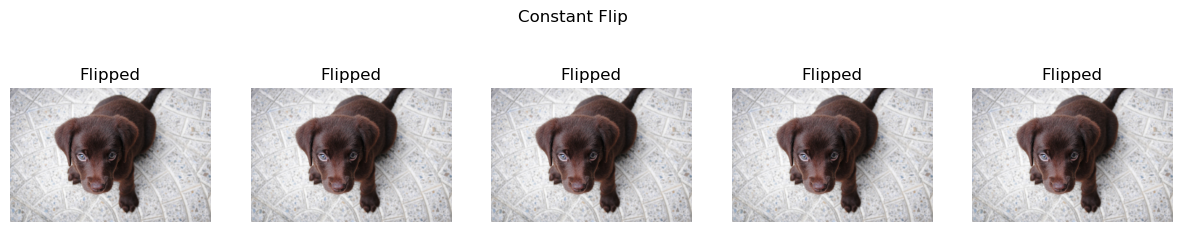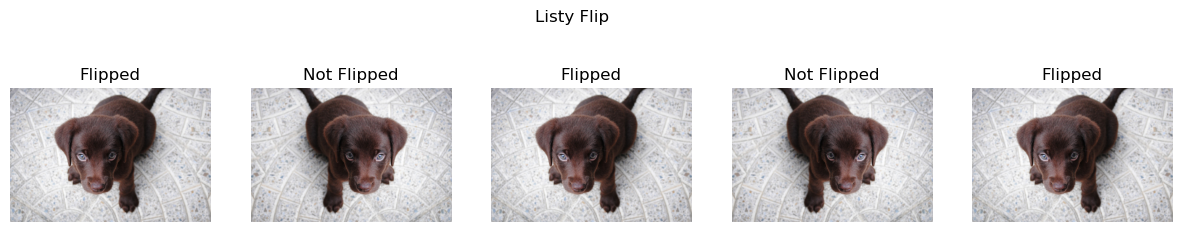``````flip = Flip(p=1.)
t = _pnt2tensor([[1,0], [2,1]], (3,3))

y = flip(TensorImage(t[None,None]), split_idx=0)
test_eq(y, _pnt2tensor([[1,0], [0,1]], (3,3))[None,None])

pnts = TensorPoint((tensor([[1.,0.], [2,1]]) -1)[None])
test_eq(flip(pnts, split_idx=0), tensor([[[1.,0.], [0,1]]]) -1)

bbox = TensorBBox(((tensor([[1.,0., 2.,1]]) -1)[None]))
test_eq(flip(bbox, split_idx=0), tensor([[[0.,0., 1.,1.]]]) -1)``````

source

### DeterministicDraw

`` DeterministicDraw (vals)``

Initialize self. See help(type(self)) for accurate signature.

``````t =  _batch_ex(8)
draw = DeterministicDraw(list(range(8)))
for i in range(15): test_eq(draw(t), torch.zeros(8)+(i%8))``````

source

### DeterministicFlip

`````` DeterministicFlip (size:int|tuple=None, mode:str='bilinear',

Flip the batch every other call

Type Default Details
size int | tuple None Output size, duplicated if one value is specified
mode str bilinear PyTorch `F.grid_sample` interpolation
pad_mode str reflection A `PadMode`
align_corners bool True PyTorch `F.grid_sample` align_corners
kwargs

Next we loop through multiple batches of the example images. DeterministicFlip will first not flip the images, and then on the next batch it will flip the images.

``````b = _batch_ex(2)
dih = DeterministicFlip()
for i,flipped in enumerate(['Not Flipped','Flipped']*2):
show_images(dih(b),suptitle=f'Batch {i}',titles=[flipped]*2)``````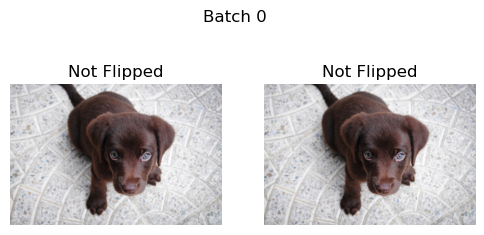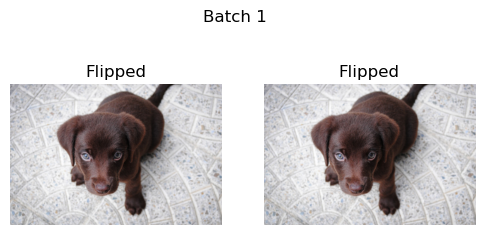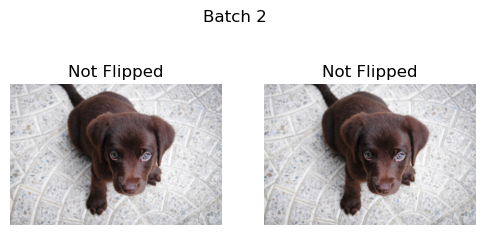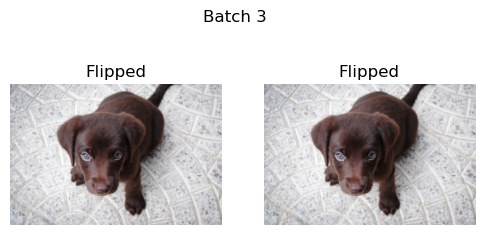Since we are working with squares and rectangles, we can think of dihedral flips as flips across the horizontal, vertical, and diagonal and their combinations. Remember though that rectangles are not symmetrical across their diagonal, so this will effectively cropping parts of rectangles.

source

### dihedral_mat

Return a random dihedral matrix

source

### Dihedral

`````` Dihedral (p=0.5, draw:int|MutableSequence|callable=None,
size:int|tuple=None, mode:str='bilinear',

Apply a random dihedral transformation to a batch of images with a probability `p`

Type Default Details
p float 0.5 Probability of applying dihedral
draw int | MutableSequence | callable None Custom dihedrals instead of random
size int | tuple None Output size, duplicated if one value is specified
mode str bilinear PyTorch `F.grid_sample` interpolation
pad_mode str reflection A `PadMode`
batch bool False Apply identical dihedral to entire batch
align_corners bool True PyTorch `F.grid_sample` align_corners

Calls `@patch`’d `dihedral_batch` behaviors for `TensorImage`, `TensorMask`, `TensorPoint`, and `TensorBBox`

`draw` can be specified if you want to customize which flip is picked when the transform is applied (default is a random number between 0 and 7). It can be an integer between 0 and 7, a list of such integers (which then should have a length equal to or greater than the size of the batch) or a callable that returns a long tensor between 0 and 7.

``````with no_random():
imgs = _batch_ex(5)
deflt = Dihedral()
const = Dihedral(p=1.,draw=1) #same as flip_batch
listy = Dihedral(p=1.,draw=[0,1,2,3,4]) #completely manual!!!
funct = Dihedral(draw=lambda x: torch.randint(0,8,(x.size(0),))) #same as default

show_images( deflt(imgs) ,suptitle='Default Flips',titles=[i for i in range(imgs.size(0))])
show_images( const(imgs) ,suptitle='Constant Horizontal Flip',titles=[f'Flip 1' for i in [0,1,1,1,1]])
show_images( listy(imgs) ,suptitle='Manual Listy Flips',titles=[f'Flip {i}' for i in [0,1,2,3,4]]) #manually specified, not random!
show_images( funct(imgs) ,suptitle='Default Functional Flips',titles=[i for i in range(imgs.size(0))]) #same as default``````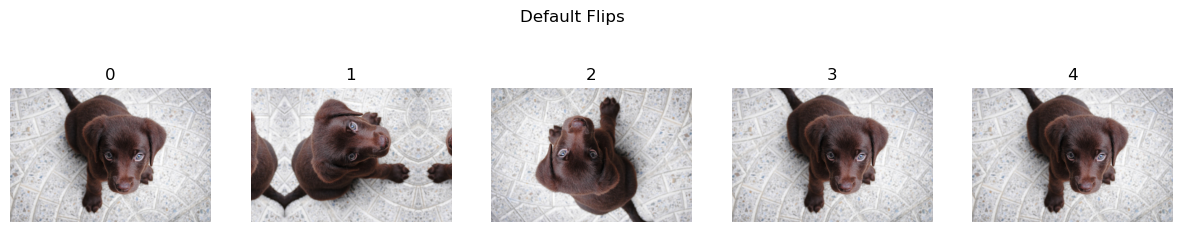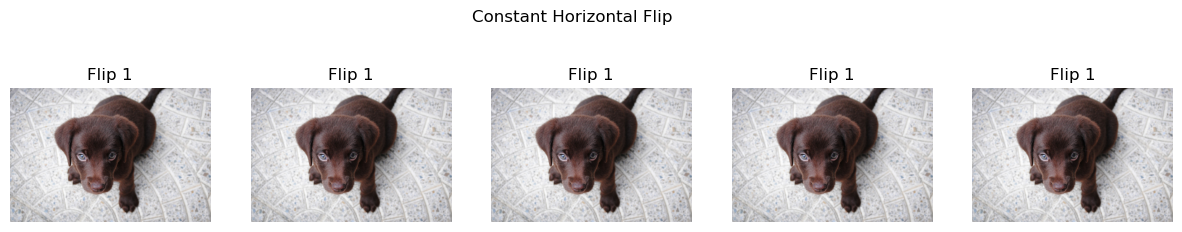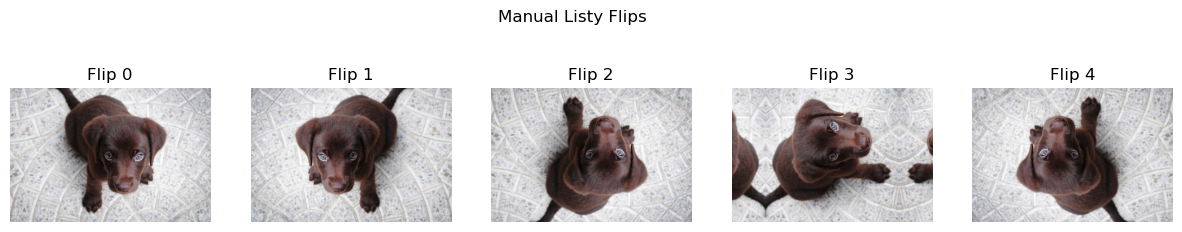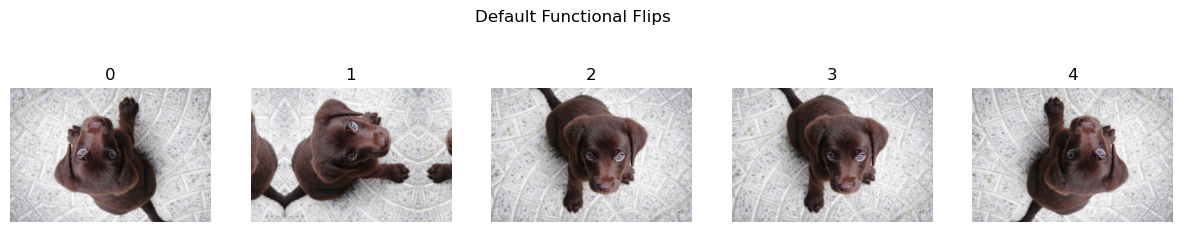source

### DeterministicDihedral

`````` DeterministicDihedral (size:int|tuple=None, mode:str='bilinear',

Apply a random dihedral transformation to a batch of images with a probability `p`

Type Default Details
size int | tuple None Output size, duplicated if one value is specified
mode str bilinear PyTorch `F.grid_sample` interpolation
pad_mode str reflection A `PadMode`
align_corners NoneType None PyTorch `F.grid_sample` align_corners

`DeterministicDihedral` guarantees that the first call will not be flipped, then the following call will be flip in a deterministic order. After all 7 possible dihedral flips the pattern will reset to the unflipped version. If we were to do this on a batch size of one it would look like this:

``````t = _batch_ex(10)
dih = DeterministicDihedral()
_,axs = plt.subplots(2,5, figsize=(14,6))
for i,ax in enumerate(axs.flatten()):
y = dih(t)
show_image(y, ctx=ax, title=f'Batch {i}')``````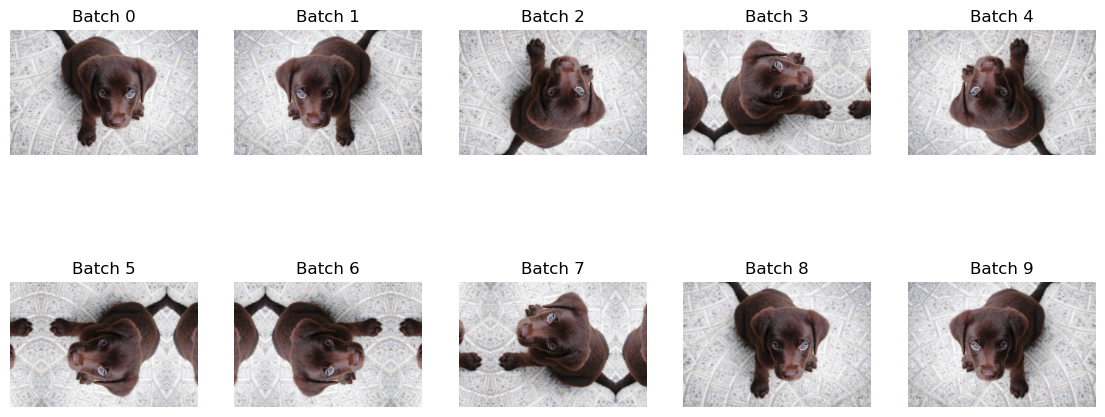source

### rotate_mat

Return a random rotation matrix with `max_deg` and `p`

source

### Rotate

`````` Rotate (max_deg:int=10, p:float=0.5,
draw:int|MutableSequence|callable=None, size:int|tuple=None,
align_corners:bool=True, batch:bool=False)``````

Apply a random rotation of at most `max_deg` with probability `p` to a batch of images

Type Default Details
max_deg int 10 Maximum degree of rotation
p float 0.5 Probability of applying rotate
draw int | MutableSequence | callable None Custom rotates instead of random
size int | tuple None Output size, duplicated if one value is specified
mode str bilinear PyTorch `F.grid_sample` interpolation
pad_mode str reflection A `PadMode`
align_corners bool True PyTorch `F.grid_sample` align_corners
batch bool False Apply identical rotate to entire batch

Calls `@patch`’d `rotate` behaviors for `TensorImage`, `TensorMask`, `TensorPoint`, and `TensorBBox`

`draw` can be specified if you want to customize which angle is picked when the transform is applied (default is a random float between `-max_deg` and `max_deg`). It can be a float, a list of floats (which then should have a length equal to or greater than the size of the batch) or a callable that returns a float tensor.

Rotate by default can only rotate 10 degrees, which makes the changes harder to see. This is usually combined with either `flip` or `dihedral`, which make much larger changes by default. A rotate of 180 degrees is the same as a vertical flip for example.

``````with no_random():
thetas = [-30,-15,0,15,30]
imgs = _batch_ex(5)
deflt = Rotate()
const = Rotate(p=1.,draw=180) #same as a vertical flip
listy = Rotate(p=1.,draw=[-30,-15,0,15,30]) #completely manual!!!
funct = Rotate(draw=lambda x: x.new_empty(x.size(0)).uniform_(-10, 10)) #same as default

show_images( deflt(imgs) ,suptitle='Default Rotate, notice the small rotation',titles=[i for i in range(imgs.size(0))])
show_images( const(imgs) ,suptitle='Constant 180 Rotate',titles=[f'180 Degrees' for i in range(imgs.size(0))])
#manually specified, not random!
show_images( listy(imgs) ,suptitle='Manual List Rotate',titles=[f'{i} Degrees' for i in [-30,-15,0,15,30]])
#same as default
show_images( funct(imgs) ,suptitle='Default Functional Rotate',titles=[i for i in range(imgs.size(0))])``````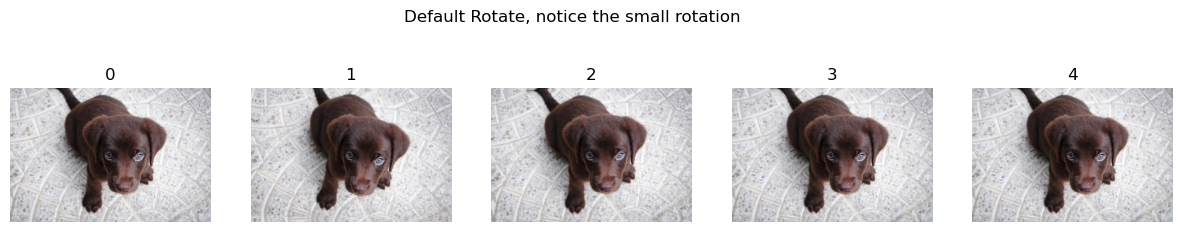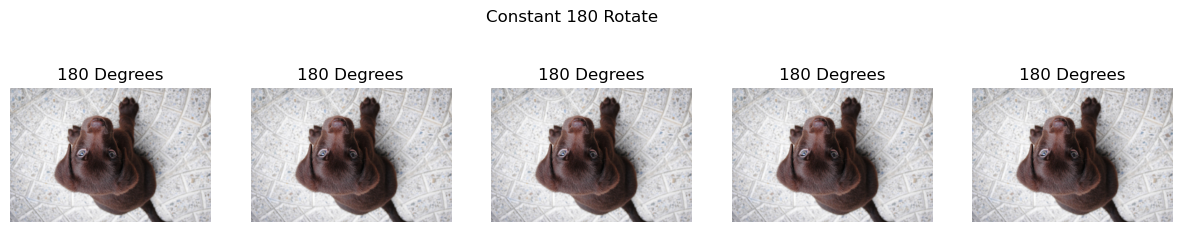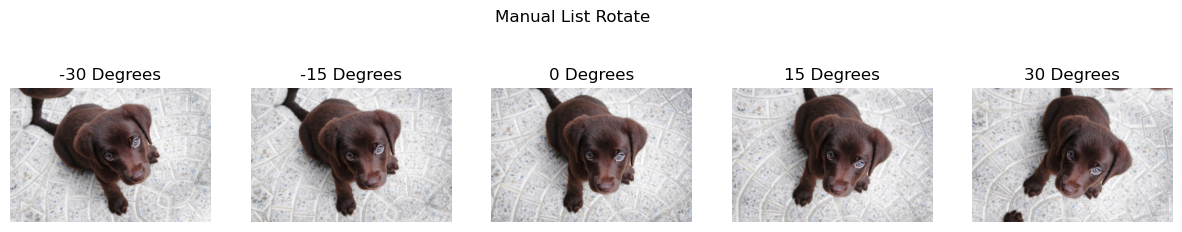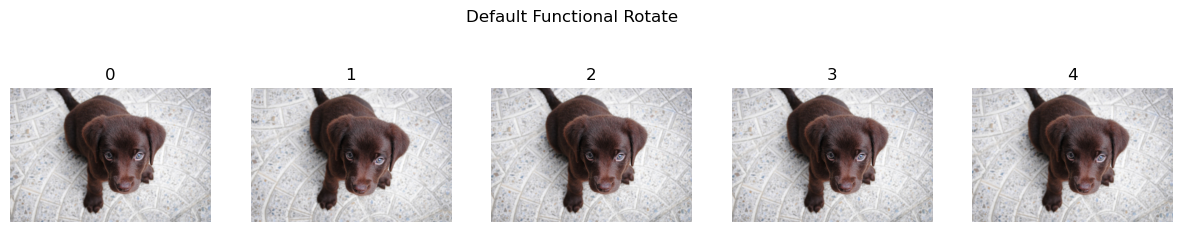source

### zoom_mat

Return a random zoom matrix with `max_zoom` and `p`

source

### Zoom

`````` Zoom (min_zoom:float=1.0, max_zoom:float=1.1, p:float=0.5,
draw:float|MutableSequence|callable=None,
draw_x:float|MutableSequence|callable=None,
draw_y:float|MutableSequence|callable=None, size:int|tuple=None,
align_corners=True)``````

Apply a random zoom of at most `max_zoom` with probability `p` to a batch of images

Type Default Details
min_zoom float 1.0 Minimum zoom
max_zoom float 1.1 Maximum zoom
p float 0.5 Probability of applying zoom
draw float | MutableSequence | callable None User defined scale of the zoom
draw_x float | MutableSequence | callable None User defined center of the zoom in x
draw_y float | MutableSequence | callable None User defined center of the zoom in y
size int | tuple None Output size, duplicated if one value is specified
mode str bilinear PyTorch `F.grid_sample` interpolation
pad_mode str reflection A `PadMode`
batch bool False Apply identical zoom to entire batch
align_corners bool True PyTorch `F.grid_sample` align_corners

Calls `@patch`’d `zoom` behaviors for `TensorImage`, `TensorMask`, `TensorPoint`, and `TensorBBox`

`draw`, `draw_x` and `draw_y` can be specified if you want to customize which scale and center are picked when the transform is applied (default is a random float between 1 and `max_zoom` for the first, between 0 and 1 for the last two). Each can be a float, a list of floats (which then should have a length equal to or greater than the size of the batch) or a callable that returns a float tensor.

`draw_x` and `draw_y` are expected to be the position of the center in pct, 0 meaning the most left/top possible and 1 meaning the most right/bottom possible.

Note: By default Zooms are rather small.

``````with no_random():
scales = [0.8, 1., 1.1, 1.25, 1.5]
imgs = _batch_ex(5)
deflt = Zoom()
const = Zoom(p=1., draw=1.5) #'Constant scale and different random centers'
listy = Zoom(p=1.,draw=scales,draw_x=0.5, draw_y=0.5) #completely manual scales, constant center
funct = Zoom(draw=lambda x: x.new_empty(x.size(0)).uniform_(1., 1.1)) #same as default

show_images( deflt(imgs) ,suptitle='Default Zoom, note the small zooming', titles=[i for i in range(imgs.size(0))])
show_images( const(imgs) ,suptitle='Constant Scale, Valiable Position', titles=[f'Scale 1.5x' for i in range(imgs.size(0))])
show_images( listy(imgs) ,suptitle='Manual Listy Scale, Centered', titles=[f'Scale {i}x' for i in scales])
show_images( funct(imgs) ,suptitle='Default Functional Zoom', titles=[i for i in range(imgs.size(0))]) #same as default``````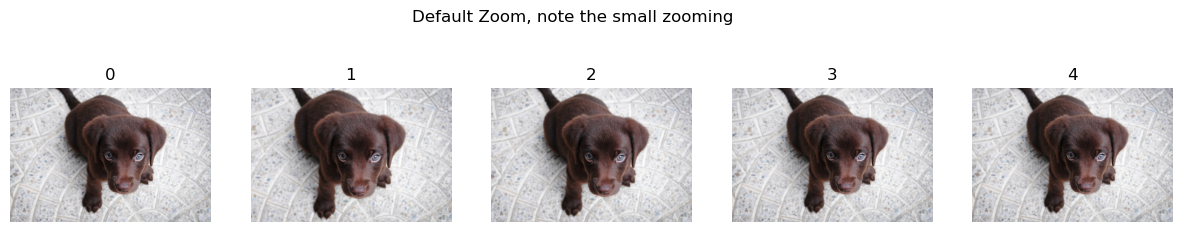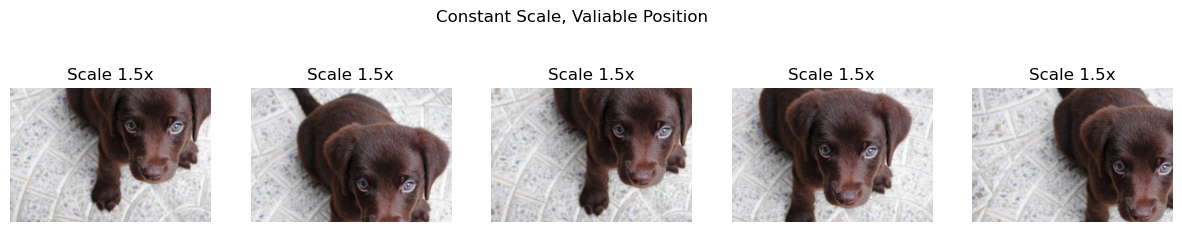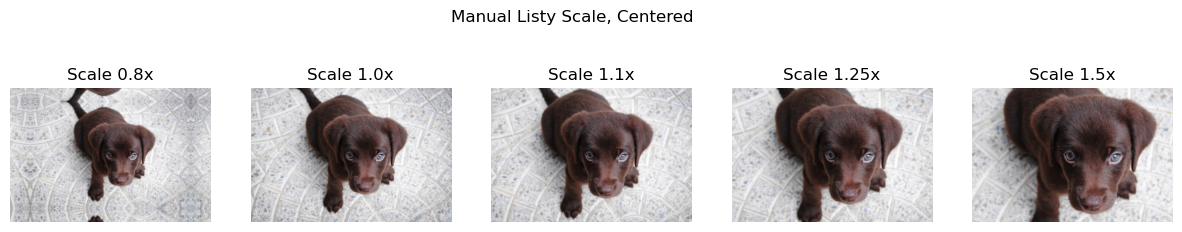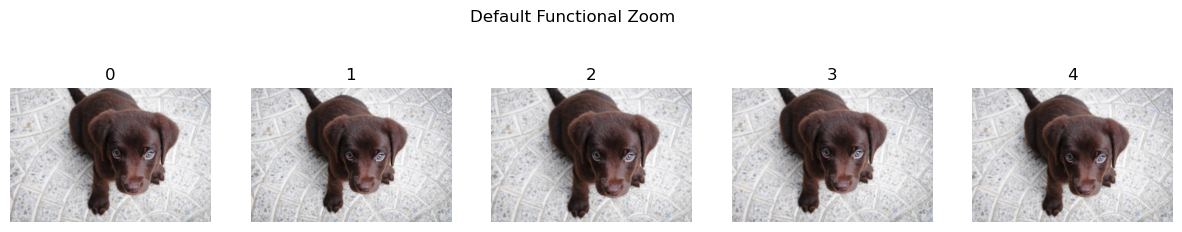source

### find_coeffs

`` find_coeffs (p1:torch.Tensor, p2:torch.Tensor)``

Find coefficients for warp tfm from `p1` to `p2`

Type Details
p1 Tensor Original points
p2 Tensor Target points

source

### apply_perspective

`` apply_perspective (coords:torch.Tensor, coeffs:torch.Tensor)``

Apply perspective tranform on `coords` with `coeffs`

Type Details
coords Tensor Original coordinates
coeffs Tensor Warping transformation matrice

source

### Warp

`````` Warp (magnitude:float=0.2, p:float=0.5,
draw_x:float|MutableSequence|callable=None,
draw_y:float|MutableSequence|callable=None, size:int|tuple=None,
align_corners:bool=True)``````

Apply perspective warping with `magnitude` and `p` on a batch of matrices

Type Default Details
magnitude float 0.2 The default warping magnitude
p float 0.5 Probability of applying warp
draw_x float | MutableSequence | callable None User defined warping magnitude in x
draw_y float | MutableSequence | callable None User defined warping magnitude in y
size int | tuple None Output size, duplicated if one value is specified
mode str bilinear PyTorch `F.grid_sample` interpolation
pad_mode str reflection A `PadMode`
batch bool False Apply identical warp to entire batch
align_corners bool True PyTorch `F.grid_sample` align_corners

Calls `@patch`’d `warp` behaviors for `TensorImage`, `TensorMask`, `TensorPoint`, and `TensorBBox`

`draw_x` and `draw_y` can be specified if you want to customize the magnitudes that are picked when the transform is applied (default is a random float between `-magnitude` and `magnitude`. Each can be a float, a list of floats (which then should have a length equal to or greater than the size of the batch) or a callable that returns a float tensor.

``````scales = [-0.4, -0.2, 0., 0.2, 0.4]
imgs=_batch_ex(5)
vert_warp = Warp(p=1., draw_y=scales, draw_x=0.)
horz_warp = Warp(p=1., draw_x=scales, draw_y=0.)
show_images( vert_warp(imgs) ,suptitle='Vertical warping', titles=[f'magnitude {i}' for i in scales])
show_images( horz_warp(imgs) ,suptitle='Horizontal warping', titles=[f'magnitude {i}' for i in scales])``````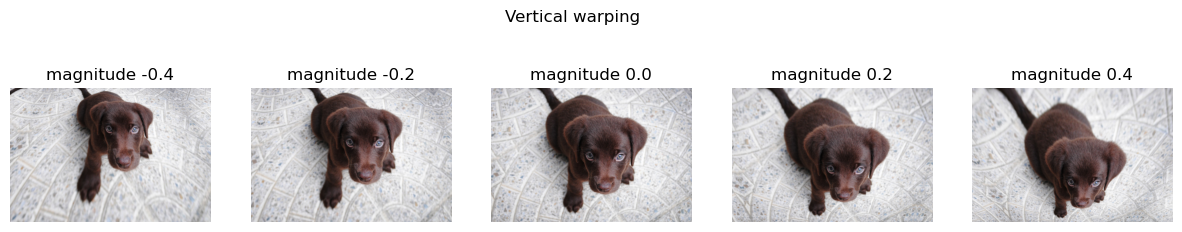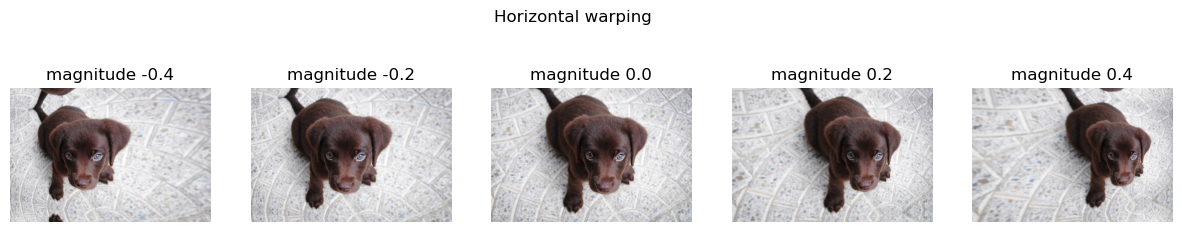## Lighting transforms

Lighting transforms are transforms that effect how light is represented in an image. These don’t change the location of the object like previous transforms, but instead simulate how light could change in a scene. The simclr paper evaluates these transforms against other transforms for their use case of self-supurved image classification, note they use “color” and “color distortion” to refer to a combination of these transforms.

source

### TensorImage.lighting

`` TensorImage.lighting (x:fastai.torch_core.TensorImage, func)``

Most lighting transforms work better in “logit space”, as we do not want to blowout the image by going over maximum or minimum brightness. Taking the sigmoid of the logit allows us to get back to “linear space.”

``````x=TensorImage(torch.tensor([.01* i for i in range(0,101)]))
f_lin= lambda x:(2*(x-0.5)+0.5).clamp(0,1) #blue line
f_log= lambda x:2*x #red line
plt.plot(x,f_lin(x),'b',x,x.lighting(f_log),'r');``````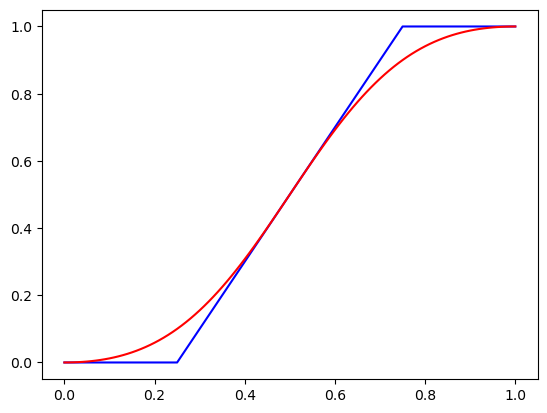The above graph shows the results of doing a contrast transformation in both linear and logit space. Notice how the blue linear plot has to be clamped, and we have lost information on how large 0.0 is by comparision to 0.2. While in the red plot the values curve, so we keep this relative information.

First we create a general `SpaceTfm`. This allows us compose multiple transforms together, so that we only have to convert to a space once, before doing multiple transforms. The `space_fn` must convert from rgb to a space, apply a function, and then convert back to rgb. `fs` should be list-like, and contain a functions that will be composed together.

source

### SpaceTfm

`` SpaceTfm (fs:callable|MutableSequence, space_fn:callable, **kwargs)``

Apply `fs` to the logits

Type Details
fs callable | MutableSequence Transformation functions applying in a space
space_fn callable Function converting rgb to a space and back to rgb after appying `fs`
kwargs

`LightingTfm` is a `SpaceTfm` that uses `TensorImage.lighting` to convert to logit space. Use this to limit images loosing detail when they become very dark or bright.

source

### LightingTfm

`` LightingTfm (fs:callable|MutableSequence, **kwargs)``

Apply `fs` to the logits

Type Details
fs callable | MutableSequence Transformation functions applying in logit space,
kwargs

Brightness refers to the amount of light on a scene. This can be zero in which the image is completely black or one where the image is completely white. This may be especially useful if you expect your dataset to have over or under exposed images.

source

### Brightness

`````` Brightness (max_lighting:float=0.2, p:float=0.75,
draw:float|MutableSequence|callable=None, batch=False)``````

Apply `fs` to the logits

Type Default Details
max_lighting float 0.2 Maximum scale of changing brightness
p float 0.75 Probability of appying transformation
draw float | MutableSequence | callable None User defined behavior of batch transformation
batch bool False Apply identical brightness to entire batch

Calls `@patch`’d `brightness` behaviors for `TensorImage`

`draw` can be specified if you want to customize the magnitude that is picked when the transform is applied (default is a random float between `-0.5*(1-max_lighting)` and `0.5*(1+max_lighting)`. Each can be a float, a list of floats (which then should have a length equal to or greater than the size of the batch) or a callable that returns a float tensor.

``````scales = [0.1, 0.3, 0.5, 0.7, 0.9]
y = _batch_ex(5).brightness(draw=scales, p=1.)
fig,axs = plt.subplots(1,5, figsize=(15,3))
for i,ax in enumerate(axs.flatten()):
show_image(y[i], ctx=ax, title=f'scale {scales[i]}')``````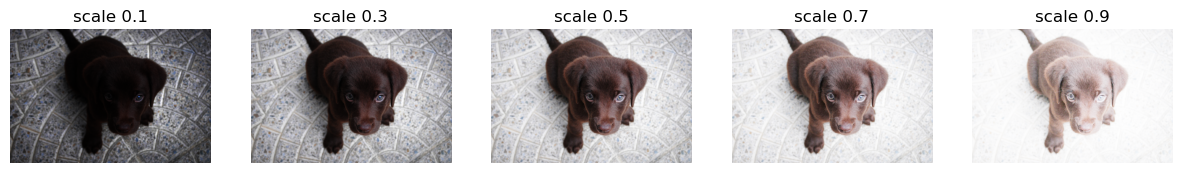Contrast pushes pixels to either the maximum or minimum values. The minimum value for contrast is a solid gray image. As an example take a picture of a bright light source in a dark room. Your eyes should be able to see some detail in the room, but the photo taken should instead have much higher contrast, with all of the detail in the background missing to the darkness. This is one example of what this transform can help simulate.

source

### Contrast

`````` Contrast (max_lighting=0.2, p=0.75,
draw:float|MutableSequence|callable=None, batch=False)``````

Apply change in contrast of `max_lighting` to batch of images with probability `p`.

Type Default Details
max_lighting float 0.2 Maximum scale of changing contrast
p float 0.75 Probability of appying transformation
draw float | MutableSequence | callable None User defined behavior of batch transformation
batch bool False

Calls `@patch`’d `contrast` behaviors for `TensorImage`

`draw` can be specified if you want to customize the magnitude that is picked when the transform is applied (default is a random float taken with the log uniform distribution between `(1-max_lighting)` and `1/(1-max_lighting)`. Each can be a float, a list of floats (which then should have a length equal to or greater than the size of the batch) or a callable that returns a float tensor.

``````scales = [0.65, 0.8, 1., 1.25, 1.55]
y = _batch_ex(5).contrast(p=1., draw=scales)
fig,axs = plt.subplots(1,5, figsize=(15,3))
for i,ax in enumerate(axs.flatten()): show_image(y[i], ctx=ax, title=f'scale {scales[i]}')``````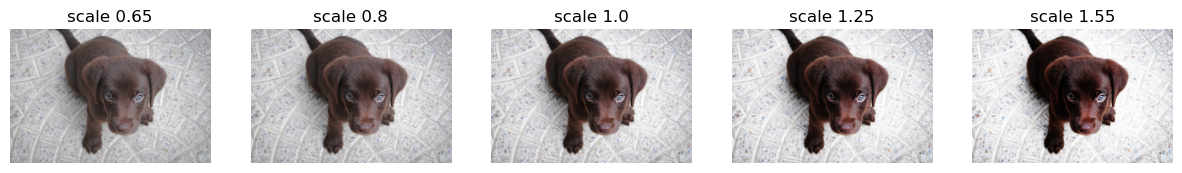source

### grayscale

`` grayscale (x)``

Tensor to grayscale tensor. Uses the ITU-R 601-2 luma transform.

The above is just one way to convert to grayscale. We chose this one because it was fast. Notice that the sum of the weight of each channel is 1.

``f'{sum([0.2989,0.5870,0.1140]):.3f}'``
``'1.000'``

source

### Saturation

`````` Saturation (max_lighting:float=0.2, p:float=0.75,
draw:float|MutableSequence|callable=None, batch:bool=False)``````

Apply change in saturation of `max_lighting` to batch of images with probability `p`.

Type Default Details
max_lighting float 0.2 Maximum scale of changing brightness
p float 0.75 Probability of appying transformation
draw float | MutableSequence | callable None User defined behavior of batch transformation
batch bool False Apply identical saturation to entire batch

Calls `@patch`’d `saturation` behaviors for `TensorImage`

``````scales = [0., 0.5, 1., 1.5, 2.0]
y = _batch_ex(5).saturation(p=1., draw=scales)
fig,axs = plt.subplots(1,5, figsize=(15,3))
for i,ax in enumerate(axs.flatten()): show_image(y[i], ctx=ax, title=f'scale {scales[i]}')``````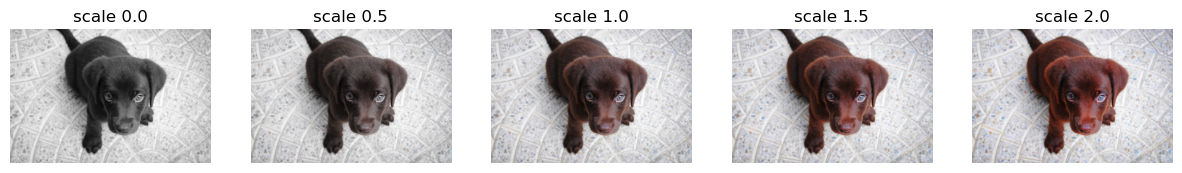Saturation controls the amount of color in the image, but not the lightness or darkness of an image. If has no effect on neutral colors such as whites,grays and blacks. At zero saturation you actually get a grayscale image. Pushing saturation past one causes more neutral colors to take on any underlying chromatic color.

`rgb2hsv`, and `hsv2rgb` are utilities for converting to and from hsv space. Hsv space stands for hue,saturation, and value space. This allows us to more easily perform certain transforms.

``torch.max(tensor().as_subclass(TensorBase), dim=0)``
``````torch.return_types.max(
values=TensorBase(1),
indices=TensorBase(0))``````

source

### rgb2hsv

`` rgb2hsv (img:torch.Tensor)``

Converts a RGB image to an HSV image. Note: Will not work on logit space images.

Type Details
img Tensor Batch of images `Tensor`in RGB

source

### hsv2rgb

`` hsv2rgb (img:torch.Tensor)``

Converts a HSV image to an RGB image.

Type Details
img Tensor Batch of images `Tensor in HSV`

Very similar to `lighting` which is done in logit space, hsv transforms are done in hsv space. We can compose any transforms that are done in hsv space.

source

### HSVTfm

`` HSVTfm (fs, **kwargs)``

Apply `fs` to the images in HSV space

Calls `@patch`’d `hsv` behaviors for `TensorImage`

``````fig,axs=plt.subplots(figsize=(20, 4),ncols=5)
axs.set_ylabel('Hue')
for ax in axs:
ax.set_xlabel('Saturation')
ax.set_yticklabels([])
ax.set_xticklabels([])

hsvs=torch.stack([torch.arange(0,2.1,0.01)[:,None].repeat(1,210),
torch.arange(0,1.05,0.005)[None].repeat(210,1),
torch.ones([210,210])])[None]
for ax,i in zip(axs,range(0,5)):
if i>0: hsvs[:,2].mul_(0.80)
ax.set_title('V='+'%.1f' %0.8**i)
ax.imshow(hsv2rgb(hsvs).permute(1,2,0))``````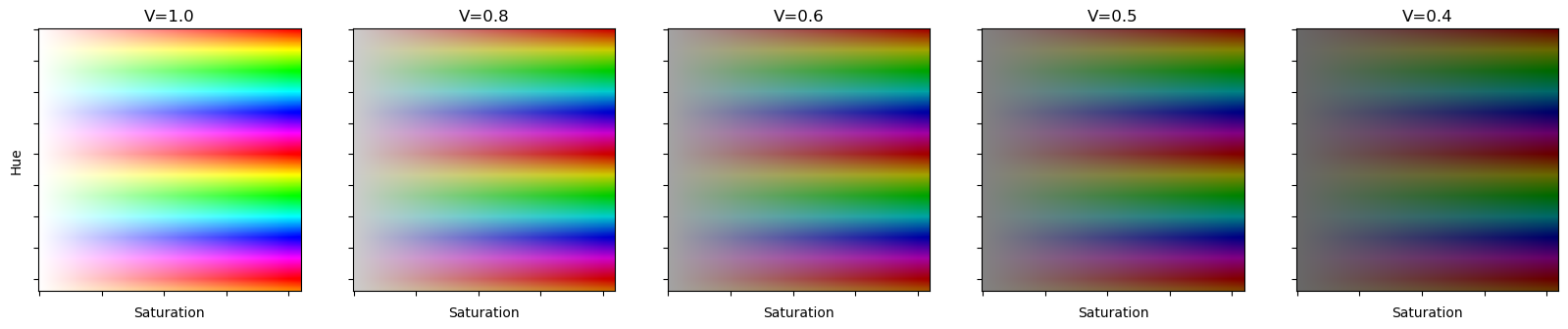For the Hue transform we are using hsv space instead of logit space. HSV stands for hue,saturation and value. Hue in hsv space just cycles through colors of the rainbow. Notices how there is no maximum, because the colors just repeat.

Above are some examples of Hue(H) and Saturation(S) at various Values(V). One property of note in HSV space is that V controls the color you get at minimum saturation when in HSV space.

source

### Hue

`````` Hue (max_hue:float=0.1, p:float=0.75,
draw:float|MutableSequence|callable=None, batch=False)``````

Apply change in hue of `max_hue` to batch of images with probability `p`.

Type Default Details
max_hue float 0.1 Maximum scale of changing Hue
p float 0.75 Probability of appying transformation
draw float | MutableSequence | callable None User defined behavior of batch transformation
batch bool False Apply identical Hue to entire batch

Calls `@patch`’d `hue` behaviors for `TensorImage`

``````scales = [0.5, 0.75, 1., 1.5, 1.75]
y = _batch_ex(len(scales)).hue(p=1., draw=scales)
fig,axs = plt.subplots(1,len(scales), figsize=(15,3))
for i,ax in enumerate(axs.flatten()): show_image(y[i], ctx=ax, title=f'scale {scales[i]}')``````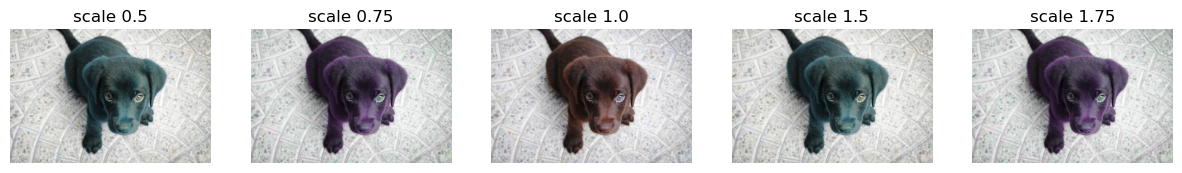## RandomErasing

Random Erasing Data Augmentation. This variant, designed by Ross Wightman, is applied to either a batch or single image tensor after it has been normalized.

source

### cutout_gaussian

`` cutout_gaussian (x:torch.Tensor, areas:list)``

Replace all `areas` in `x` with N(0,1) noise

Type Details
x Tensor Input image
areas list List of areas to cutout. Order rl,rh,cl,ch

Since this should be applied after normalization, we’ll define a helper to apply a function inside normalization.

source

### norm_apply_denorm

`````` norm_apply_denorm (x:torch.Tensor, f:<built-infunctioncallable>,
nrm:<built-infunctioncallable>)``````

Normalize `x` with `nrm`, then apply `f`, then denormalize

Type Details
x Tensor Input Image
f callable Function to apply
nrm callable Normalization transformation
``nrm = Normalize.from_stats(*imagenet_stats, cuda=False)``
``````f = partial(cutout_gaussian, areas=[(100,200,100,200),(200,300,200,300)])
show_image(norm_apply_denorm(timg, f, nrm));``````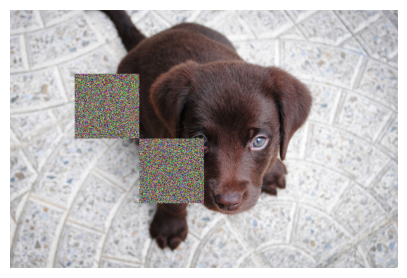source

### RandomErasing

`````` RandomErasing (p:float=0.5, sl:float=0.0, sh:float=0.3,
min_aspect:float=0.3, max_count:int=1)``````

Randomly selects a rectangle region in an image and randomizes its pixels.

Type Default Details
p float 0.5 Probability of appying Random Erasing
sl float 0.0 Minimum proportion of erased area
sh float 0.3 Maximum proportion of erased area
min_aspect float 0.3 Minimum aspect ratio of erased area
max_count int 1 Maximum number of erasing blocks per image, area per box is scaled by count
``````tfm = RandomErasing(p=1., max_count=6)

_,axs = subplots(2,3, figsize=(12,6))
f = partial(tfm, split_idx=0)
for i,ax in enumerate(axs.flatten()): show_image(norm_apply_denorm(timg, f, nrm), ctx=ax)``````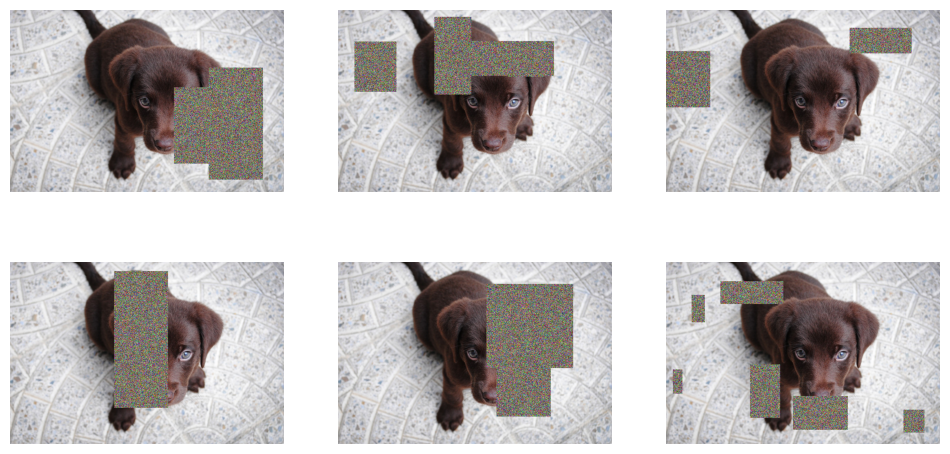``````tfm = RandomErasing(p=1., max_count=6)

_,axs = subplots(2,3, figsize=(12,6))
f = partial(tfm, split_idx=0)
for i,ax in enumerate(axs.flatten()): show_image(norm_apply_denorm(timg, f, nrm), ctx=ax)``````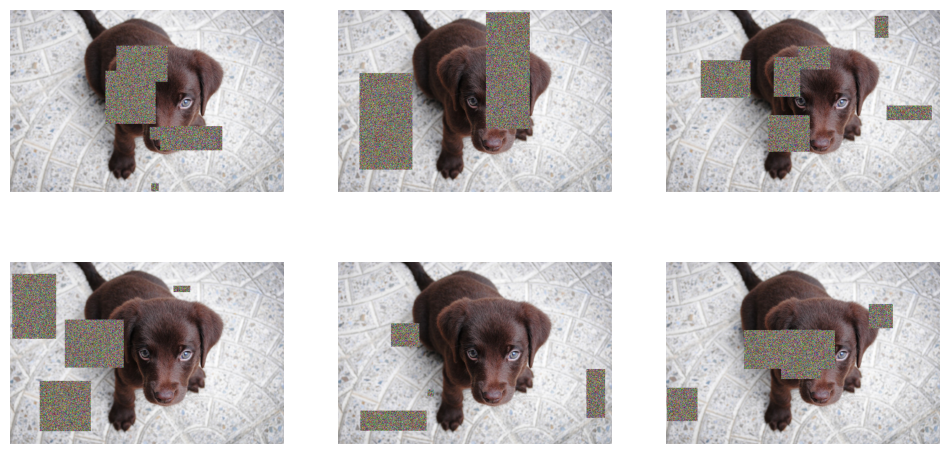``````tfm = RandomErasing(p=1., max_count=6)

_,axs = subplots(2,3, figsize=(12,6))
f = partial(tfm, split_idx=1)
for i,ax in enumerate(axs.flatten()): show_image(norm_apply_denorm(timg, f, nrm), ctx=ax)``````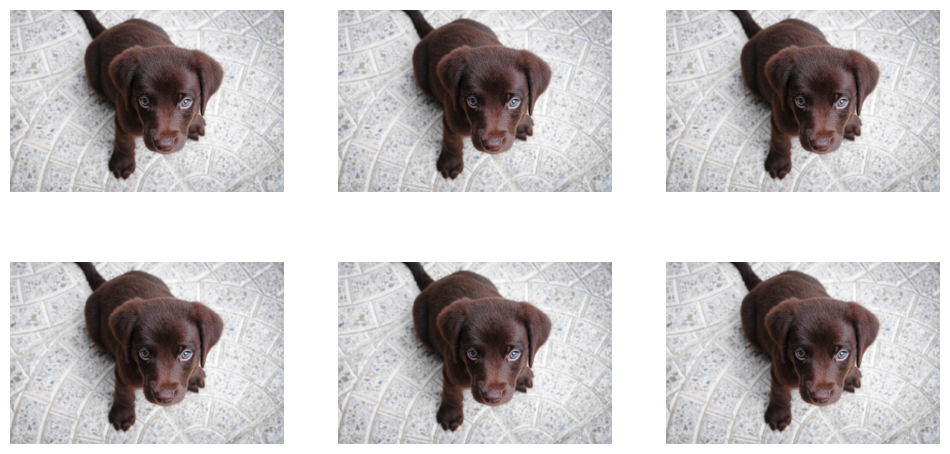## All together

source

### setup_aug_tfms

`` setup_aug_tfms (tfms)``

Go through `tfms` and combines together affine/coord or lighting transforms

``````#Affine only
tfms = [Rotate(draw=10., p=1), Zoom(draw=1.1, draw_x=0.5, draw_y=0.5, p=1.)]
comp = setup_aug_tfms([Rotate(draw=10., p=1), Zoom(draw=1.1, draw_x=0.5, draw_y=0.5, p=1.)])
test_eq(len(comp), 1)
x = torch.randn(4,3,5,5)
test_close(comp._get_affine_mat(x)[...,:2],tfms._get_affine_mat(x)[...,:2] @ tfms._get_affine_mat(x)[...,:2])
#We can't test that the ouput of comp or the composition of tfms on x is the same cause it's not (1 interpol vs 2 sp)``````
``````#Affine + lighting
tfms = [Rotate(), Zoom(), Warp(), Brightness(), Flip(), Contrast()]
comp = setup_aug_tfms(tfms)``````
``````aff_tfm,lig_tfm = comp
test_eq(len(aff_tfm.aff_fs+aff_tfm.coord_fs+comp.fs), 6)
test_eq(len(aff_tfm.aff_fs), 3)
test_eq(len(aff_tfm.coord_fs), 1)
test_eq(len(lig_tfm.fs), 2)``````

source

### aug_transforms

`````` aug_transforms (mult:float=1.0, do_flip:bool=True, flip_vert:bool=False,
max_rotate:float=10.0, min_zoom:float=1.0,
max_zoom:float=1.1, max_lighting:float=0.2,
max_warp:float=0.2, p_affine:float=0.75,
p_lighting:float=0.75, xtra_tfms:list=None,
size:int|tuple=None, mode:str='bilinear',
min_scale=1.0)``````

Utility func to easily create a list of flip, rotate, zoom, warp, lighting transforms.

Type Default Details
mult float 1.0 Multiplication applying to `max_rotate`,`max_lighting`,`max_warp`
do_flip bool True Random flipping
flip_vert bool False Flip vertically
max_rotate float 10.0 Maximum degree of rotation
min_zoom float 1.0 Minimum zoom
max_zoom float 1.1 Maximum zoom
max_lighting float 0.2 Maximum scale of changing brightness
max_warp float 0.2 Maximum value of changing warp per
p_affine float 0.75 Probability of applying affine transformation
p_lighting float 0.75 Probability of changing brightnest and contrast
xtra_tfms list None Custom Transformations
size int | tuple None Output size, duplicated if one value is specified
mode str bilinear PyTorch `F.grid_sample` interpolation
pad_mode str reflection A `PadMode`
align_corners bool True PyTorch `F.grid_sample` align_corners
batch bool False Apply identical transformation to entire batch
min_scale float 1.0 Minimum scale of the crop, in relation to image area

Random flip (or dihedral if `flip_vert=True`) with `p=0.5` is added when `do_flip=True`. With `p_affine` we apply a random rotation of `max_rotate` degrees, a random zoom between `min_zoom` and `max_zoom` and a perspective warping of `max_warp`. With `p_lighting` we apply a change in brightness and contrast of `max_lighting`. Custom `xtra_tfms` can be added. `size`, `mode` and `pad_mode` will be used for the interpolation. `max_rotate,max_lighting,max_warp` are multiplied by `mult` so you can more easily increase or decrease augmentation with a single parameter.

``````tfms = aug_transforms(pad_mode='zeros', mult=2, min_scale=0.5)
y = _batch_ex(9)
for t in tfms: y = t(y, split_idx=0)
_,axs = plt.subplots(1,3, figsize=(12,3))
for i,ax in enumerate(axs.flatten()): show_image(y[i], ctx=ax)````````````tfms = aug_transforms(pad_mode='zeros', mult=2, batch=True)
y = _batch_ex(9)
for t in tfms: y = t(y, split_idx=0)
_,axs = plt.subplots(1,3, figsize=(12,3))
for i,ax in enumerate(axs.flatten()): show_image(y[i], ctx=ax)``````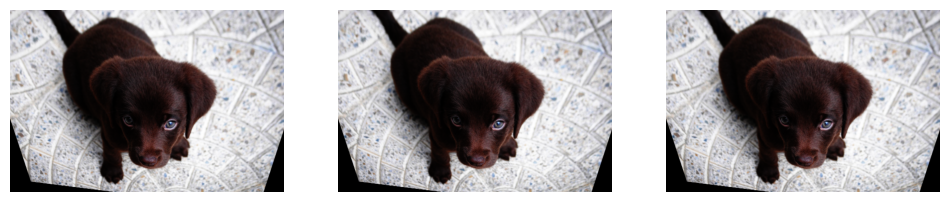## Integration tests

### Segmentation

``````camvid = untar_data(URLs.CAMVID_TINY)
fns = get_image_files(camvid/'images')
cam_fn = fns
``````cam_dsrc = Datasets([cam_fn]*10, [PILImage.create, [_cam_lbl, PILMask.create]])
cam_tdl = TfmdDL(cam_dsrc.train, after_item=ToTensor(),
after_batch=[IntToFloatTensor(), *aug_transforms()], bs=9)
cam_tdl.show_batch(max_n=9, vmin=1, vmax=30)``````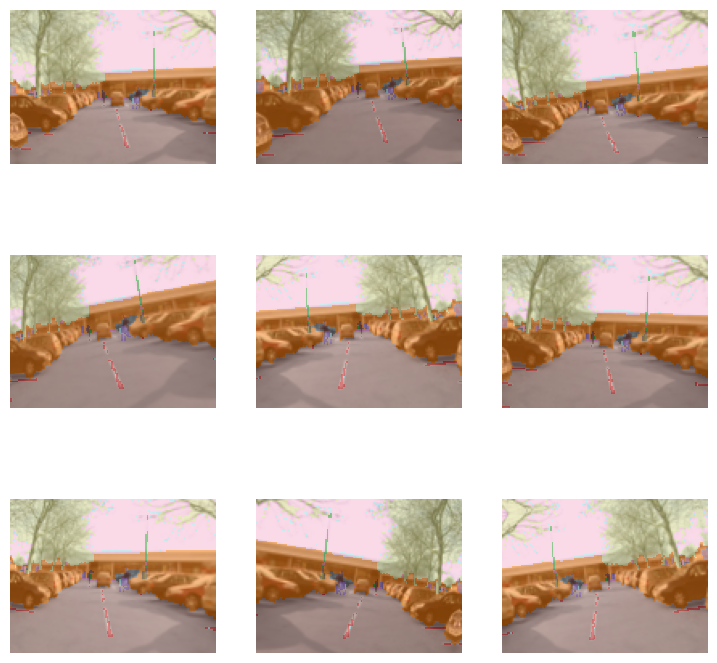### Point targets

``````mnist = untar_data(URLs.MNIST_TINY)
mnist_fn = 'images/mnist3.png'
pnts = np.array([[0,0], [0,35], [28,0], [28,35], [9, 17]])
def _pnt_lbl(fn)->None: return TensorPoint.create(pnts)``````
``````pnt_dsrc = Datasets([mnist_fn]*10, [[PILImage.create, Resize((35,28))], _pnt_lbl])
pnt_tdl = TfmdDL(pnt_dsrc.train, after_item=[PointScaler(), ToTensor()],
after_batch=[IntToFloatTensor(), *aug_transforms(max_warp=0)], bs=9)
pnt_tdl.show_batch(max_n=9)``````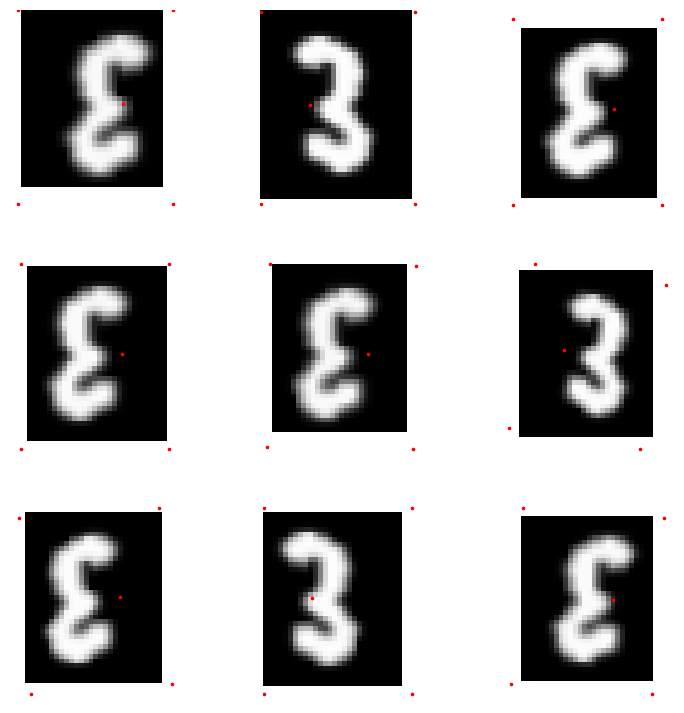### Bounding boxes

``````coco = untar_data(URLs.COCO_TINY)
images, lbl_bbox = get_annotations(coco/'train.json')
idx=2
coco_fn,bbox = coco/'train'/images[idx],lbl_bbox[idx]

def _coco_bb(x):  return TensorBBox.create(bbox)
def _coco_lbl(x): return bbox``````
``````coco_dsrc = Datasets([coco_fn]*10, [PILImage.create, [_coco_bb], [_coco_lbl, MultiCategorize(add_na=True)]], n_inp=1)
coco_tdl = TfmdDL(coco_dsrc, bs=9, after_item=[BBoxLabeler(), PointScaler(), ToTensor(), Resize(256)],
after_batch=[IntToFloatTensor(), *aug_transforms()])

coco_tdl.show_batch(max_n=9)``````